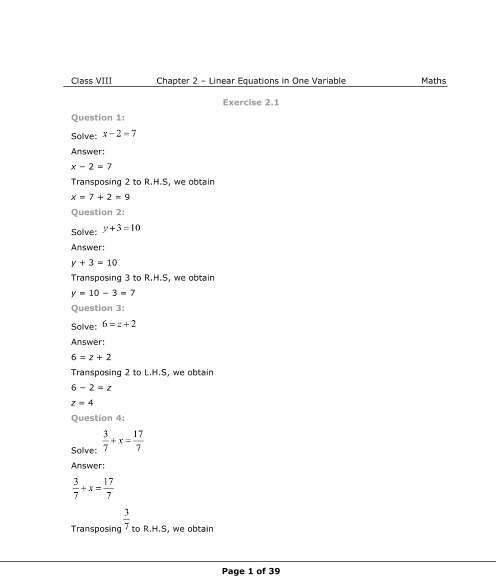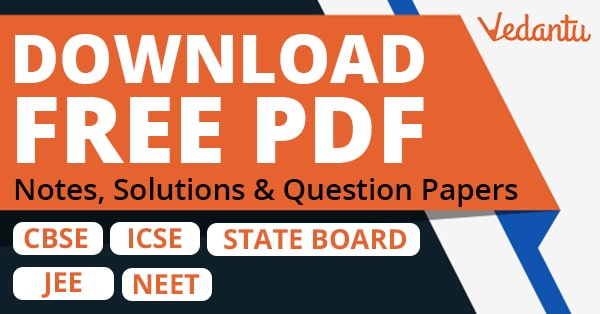# NCERT Solutions for Class 8 Maths Chapter 2 Linear Equations in One Variable (EX 2.2) Exercise 2.2

## NCERT Solutions for Class 8 Maths Chapter 2 Linear Equations in One Variable (EX 2.2) Exercise 2.2

Free PDF download of NCERT Solutions for Class 8 Maths Chapter 2 Exercise 2.2 (EX 2.2) and all chapter exercises at one place prepared by expert teacher as per NCERT (CBSE) books guidelines. Class 8 Maths Chapter 2 Linear Equations in One Variable Exercise 2.2 Questions with Solutions to help you to revise complete Syllabus and Score More marks. Register and get all exercise solutions in your emails.